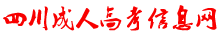﻿ 【江苏成考】专升本数学1---常微分方程-江苏成人高考信息网# 【江苏成考】专升本数学1---常微分方程G(y)=F(x)+C , y=F (x)x=Y(y)都是方程的通解, 其中G(y)=F(x)+C称为隐式(通)解。

1、齐次线性方程的解法

2、 非齐次线性方程的解法

：非齐次线性方程的通解等于对应的齐次线性方程通解与非齐次线性方程的一个特解之和.r2-2r-3=0, (r+1)(r-3)=0.

y=C1e-x+C2e3x.

——f(x)=Pm(x)elx时，二阶常系数非齐次线性微分方程(8.1)具有形如

1）与所给方程对应的齐次方程为：y¢¢-5y¢+6y=0,

Y=C1e2x+C2e3x .

2）由于l=2是特征方程的单根, 所以应设方程的特解为

y*=x(b0x+b1)e2x.

-2b0x+2b0-b1=x.

3）从而所给方程的通解为

### 江苏成考交流群### 点击加入考生微信群

#### 最新信息榜